International
Tables for
Crystallography
Volume A
Space-group symmetry
Edited by Th. Hahn

International Tables for Crystallography (2006). Vol. A, ch. 1.3, p. 6

## Section 1.3.2. Notes on symmetry elements and symmetry operations

Th. Hahna*

aInstitut für Kristallographie, Rheinisch-Westfälische Technische Hochschule, Aachen, Germany
Correspondence e-mail: hahn@xtal.rwth-aachen.de

### 1.3.2. Notes on symmetry elements and symmetry operations

| top | pdf |

• (i) Section 1.3.1contains only those symmetry elements and symmetry operations which occur in the Hermann–Mauguin symbols of point groups and space groups. Further so-called additional symmetry elements' are described in Chapter 4.1and listed in Tables 4.2.1.1and 4.3.2.1in the form of extended Hermann–Mauguin symbols'.

• (ii) The printed symbols of symmetry elements (symmetry operations), except for glide planes (glide reflections), are independent of the choice and the labelling of the basis vectors and of the origin. The symbols of glide planes (glide reflections), however, may change with a change of the basis vectors. For this reason, the possible orientations of glide planes and the glide vectors of the corresponding operations are listed explicitly in columns 2 and 3.

• (iii) In space groups, further kinds of glide planes and glide reflections (called g) occur which are not used in the Hermann–Mauguin symbols. They are listed in the space-group tables (Part 7) under Symmetry operations and in Table 4.3.2.1for the tetragonal and cubic space groups; they are explained in Sections 2.2.9and 11.1.2.

• (iv) Whereas the term symmetry operation' is well defined (cf. Section 8.1.3), the word symmetry element' is used by crystallographers in a variety of often rather loose meanings. In 1989, the International Union of Crystallography published a Nomenclature Report which first defines a geometric element' as a geometric item that allows the fixed points of a symmetry operation (after removal of any intrinsic glide or screw translation) to be located and oriented in a coordinate system. A symmetry element' then is defined as a concept with a double meaning, namely the combination of a geometric element with the set of symmetry operations having this geometric element in common (element set'). For further details and tables, see de Wolff et al. (1989)and Flack et al. (2000).

• (v) To each glide plane, infinitely many different glide reflections belong, because to each glide vector listed in column 3 any lattice translation vector parallel to the glide plane may be added; this includes centring vectors of centred cells. Each resulting vector is a glide vector of a new glide reflection but with the same plane as the geometric element. Any of these glide operations can be used as a defining operation'.

#### Examples

 (1) Glide plane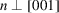: All vectors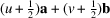are glide vectors (u, v any integers); this includes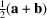,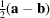,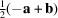,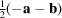. (2) Glide plane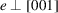in a C-centred cell: All vectors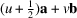and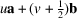are glide vectors, this includes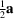and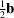(which are related by the centring vector), i.e. the glide plane e is at the same time a glide plane a and a glide plane b; for this double' glide plane e see Note (x)below. (3) Glide plane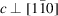in an F-centred cell: All vectors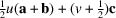are glide vectors; this includes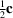and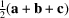, i.e. the glide plane c is at the same time a glide plane n.

• (vi) If among the infinitely many glide operations of the element set of a symmetry plane there exists one operation with glide vector zero, then this symmetry element is a mirror plane.

• (vii) Similar considerations apply to screw axes; to the screw vector defined in column 3 any lattice translation vector parallel to the screw axis may be added. Again, this includes centring vectors of centred cells.

#### Example

Screw axis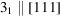in a cubic primitive cell. For the first power (right-handed screw rotation of 120°), all vectors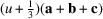are screw vectors; this includes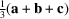,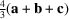,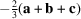. For the second power (right-handed screw rotation of 240°), all vectors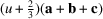are screw vectors; this includes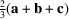,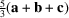;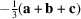. The third power corresponds to all lattice vectors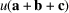.

Again, if one of the screw vectors is zero, the symmetry element is a rotation axis.

• (viii) In the space-group tables, under Symmetry operations, for rotations, screw rotations and roto-inversions, the sense of rotation' is indicated by symbols like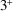,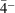etc.; this is explained in Section 11.1.2.

• (ix) The members of the following pairs of screw axes are enantiomorphic', i.e. they can be considered as a right- and a left-handed screw, respectively, with the same screw vector: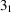,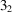;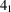,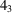;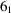,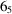;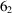,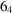. The following screw axes are neutral', i.e. they contain left- and right-handed screws with the same screw vector: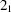;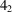;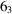.

• (x) In the third Nomenclature Report of the IUCr (de Wolff et al., 1992), two new printed symbols for glide planes were proposed: e for double' glide planes and k for transverse' glide planes.

For the e glide planes, new graphical symbols were introduced (cf. Sections 1.4.1, 1.4.2, 1.4.3and Note iv in 1.4.4); they are applied to the diagrams of the relevant space groups: Seven orthorhombic A-, C- and F-space groups, five tetragonal I-space groups, and five cubic F- and I-space groups. The e glide plane occurs only in centred cells and is defined by one plane with two perpendicular glide vectors related by a centring translation; thus, in Cmma (67), two glide operations a and b through the plane xy0 occur, their glide vectors being related by the centring vector; the symbol e removes the ambiguity between the symbols a and b.

For five space groups, the Hermann–Mauguin symbol has been modified:

Space group No.3941646768
New symbol: Aem2 Aea2 Cmce Cmme Ccce
Former symbol: Abm2 Aba2 Cmca Cmma Ccca

The new symbol is now the standard one; it is indicated in the headline of these space groups, while the former symbol is given underneath.

For the k glide planes, no new graphical symbol and no modification of a space-group symbol are proposed.

### References

Flack, H. D., Wondratschek, H., Hahn, Th. & Abrahams, S. C. (2000). Symmetry elements in space groups and point groups. Addenda to two IUCr Reports on the Nomenclature of Symmetry. Acta Cryst. A56, 96–98.
Wolff, P. M. de, Billiet, Y., Donnay, J. D. H., Fischer, W., Galiulin, R. B., Glazer, A. M., Senechal, M., Shoemaker, D. P., Wondratschek, H., Hahn, Th., Wilson, A. J. C. & Abrahams, S. C. (1989). Definition of symmetry elements in space groups and point groups. Report of the International Union of Crystallography Ad-hoc Committee on the Nomenclature of Symmetry. Acta Cryst. A45, 494–499.
Wolff, P. M. de, Billiet, Y., Donnay, J. D. H., Fischer, W., Galiulin, R. B., Glazer, A. M., Hahn, Th., Senechal, M., Shoemaker, D. P., Wondratschek, H., Wilson, A. J. C. & Abrahams, S. C. (1992). Symbols for symmetry elements and symmetry operations. Final Report of the International Union of Crystallography Ad-hoc Committee on the Nomenclature of Symmetry. Acta Cryst. A48, 727–732.